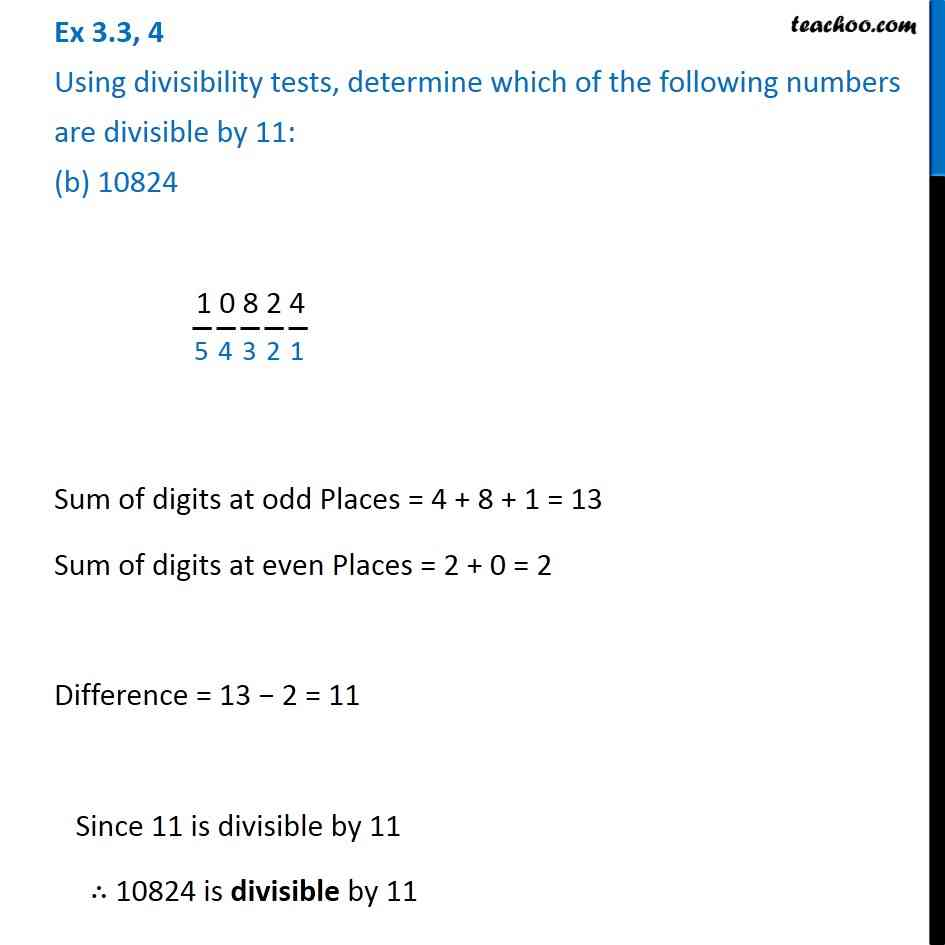Ex 3.3

Chapter 3 Class 6 Playing with Numbers
Serial order wiseLearn in your speed, with individual attention - Teachoo Maths 1-on-1 Class

### Transcript

Ex 3.3, 4 Using divisibility tests, determine which of the following numbers are divisible by 11: (b) 10824 1 0 8 2 4 Sum of digits at odd Places = 4 + 8 + 1 = 13 Sum of digits at even Places = 2 + 0 = 2 Difference = 13 − 2 = 11 Since 11 is divisible by 11 ∴ 10824 is divisible by 11